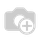# [Odoo 12] How to save updates made on One2many field through onchange method?

Dear Community,

I'm a new comer on Odoo (v12). It's been few days and i cannot resolve my issue.

I have a 3 models (equipments, functions and states)

On a specific function, i can have many equipments, 1 is deployed, the other ones are only spares. I want to switch them using only the checkbox (deploy) from the function view.

day d:

function A

equipment A1 (name)    deployed (state) True (deploy)

equipment A2 (name)    spare (state) False (deploy)

day d+x: the agent go on the function view, click modify and check the new equipment to be deployed (A2)

equipment A1 (name)    spare (state) False (deploy)

equipment A2 (name)    deployed (state) True (deploy)

`class States(models.Model):`
`_name = 'states'`
`name = fields.Char(string="State")`

`class Equipments(models.Model):`
`_name = 'equipments'`
`_order = 'deploy'`
`name = fields.Char(string="Name", required=True)`
`function = fields.Many2one('functions', string="Function")`
`state = fields.Many2one('states', string="State")`
`deploy = fields.Boolean(string="Deploy")`

`class Functions(models.Model):`
`_name = 'functions'`
`name = fields.Char(string="Name", required=True)`
`equipment_ids = fields.One2many('equipments','function')`
`@api.onchange('equipment_ids','equipment_ids.deploy')`
`def _deploy_equipment(self):`
`eqpt_ids = []`
`state_deploy = self.env['states'].search([('name','=','Deployed')])`
`state_spare = self.env['states'].search([('name','=','Spare')])`
`for eqpt in self.equipment_ids:`
`if eqpt.state == state_deploy:`
`eqpt_ids.append((1, eqpt.id, {'deploy': False, 'state': state_spare}))`
`elif eqpt.state == state_spare:`
`eqpt_ids.append((1, eqpt.id, {'deploy': True, 'state': state_deploy}))`
`self.update({'equipment_ids': eqpt_ids})`

It updates correctly my view, but when i'm saving, all data are rollbacked.

Any tip? Thx

1 Comment